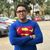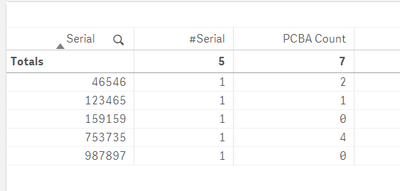# New to Qlik Sense

If you’re new to Qlik Sense, start with this Discussion Board and get up-to-speed quickly.

Announcements
cancel
Showing results for
Did you mean:Contributor III

## replase excel formula [count.plus.if]

Hello to all,

in my database I have 10 different columns where each column could contain one sting like "PCBA MB".

Normally for check if one string contain one specific value I'm use wildmatch(column1,'PCBA MB*')

but in qlik sense View, in expression, how can increase one local variable when this value it's true in diferent columns?normally in excel I use  this forumula:

=count.plus.if(BM3:CF3;"PCBA MB*")

Can I replease he formula above in qlik?

5 RepliesContributor III
Author

Hello, who can help me?

thanksContributor III

hi,

There are many ways you could do it, but if we try to create a measure in Qliksense, you can use the below method.

Firstly, we need to identify a common field across all columns, maybe like an ID.

Then create a measure withing the Qliksense app:

count({< [Column1] = {"*PCBA MB"} >}  [ID])

+

count({< [Column2] = {"*PCBA MB"} >}  [ID])

+

count({< [Column3] = {"*PCBA MB"} >}  [ID])

In the above formula, you are counting ID, in specific column, where there word PCBA MB is found.

Let me know if this works.Contributor III
Author

Hello @MohasinKhan

mmm not work perfectly becasue  my result should be:

 Serial Column 1 Column 2 Column 3 Column 4 Column 5 Column 6 Column 7 Column 8 Column 9 Column 10 PCBA Check 123465 PCBA MB other other other other other other other other other 1 987897 other other other other other other other other other other 0 46546 PCBA MB other other PCBA MB other other other other other other 2 159159 other other other other other other other other other other 0 753735 PCBA MB other other PCBA MB other other other PCBA MB other PCBA MB 4Contributor III

Hello @lukeert19

Thank you for sharing the sample, it helped me understand what was happening.

When i calculated the formula, the table was showing only those Serial numbers where we have PCBA MB.

So you need to create 2 Measures:

1. count(Serial) - name it as #Serial
2. use below formula to create the actual PCBA Check measure

count({< [Column 1] = {"*PCBA MB"} >} Serial)
+
count({< [Column 2] = {"*PCBA MB"} >} Serial)
+
count({< [Column 3] = {"*PCBA MB"} >} Serial)
+
count({< [Column 4] = {"*PCBA MB"} >} Serial)
+
count({< [Column 5] = {"*PCBA MB"} >} Serial)
+
count({< [Column 6] = {"*PCBA MB"} >} Serial)
+
count({< [Column 7] = {"*PCBA MB"} >} Serial)
+
count({< [Column 8] = {"*PCBA MB"} >} Serial)
+
count({< [Column 9] = {"*PCBA MB"} >} Serial)
+
count({< [Column 10] = {"*PCBA MB"} >} Serial)

Once i did that, I get the below results.Contributor III
Author

hello @MohasinKhan

what do you means for point one? --> count(Serial) - name it as #Serial?

I have to insert in load script something like

,count(Serial) as Serial?

and in expression I have to use the formula above?